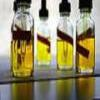#### You may also like### Mathematical Issues for Chemists

A brief outline of the mathematical issues faced by chemistry students.### Reaction Rates

Explore the possibilities for reaction rates versus concentrations with this non-linear differential equation### Catalyse That!

Can you work out how to produce the right amount of chemical in a temperature-dependent reaction?

# Spectrometry Detective

##### Age 16 to 18Challenge Level

This problem involves mathematical reasoning concerning elements of chemistry. For an introduction to mass spectrometry read our article Inspect Your Gadgets.

A diatomic gas of an element with a single stable isotope is analysed in a mass spectrometer. How many peaks will there be? How many peaks will there be if the element forming a diatomic gas has $2$ or $3$ stable isotopes?

When water is analysed in a mass spectrometer there are peaks at relative atomic mass $17$ and $18$. What chemicals do these peaks correspond to? Why are there no peaks at $1$ and $16$?

A compound is analysed and has peaks at $35, 37, 70, 72$ and $74$. What is this compound?

Another compound has peaks at $12, 13, 14, 15, 16$. What might this be? What is it definitely not?

Another compound has peaks at $14, 15, 16, 17$. What might this be? What is it definitely not?

A mixture of two chemicals is analysed and has peaks at $35, 36, 37, 38$ and $40$. What might this be? What is it definitely not?

Extension: A final compound has peaks at (from tallest to smallest) $31, 45, 29, 27, 46, 43, 26, 30, 15, 42, 28, 19, 25, 14, 13, 41, 47, 44, 17, 24, 18, 33, 12$. Can you suggest a likely candidate for the compound? What could the various peaks correspond to?

Other mathematical chemistry problems can be found on the chemNRICH pages.

NOTES AND BACKGROUND
This problem gives the opportunity to practise analysing results from mass spectrometry experiments. In this important process compounds are heated and the relative mass and frequency of the resulting fragments are measured. From the mass-spectrum it is often possible to determine the chemical composition of the original compound. To do this requires a knowledge of the atomic mass and relative abundance of the isotopes of the elements. There is a strong experimental input to this process as more complex molecules, such as long hydrocarbons break into a wide variety of fragments which complicated the analysis.

Source of data:

Mass Spectra by NIST Mass Spec Data Center, S.E. Stein, director, in NIST Chemistry WebBook, NIST Standard Reference Database Number 69 , Eds. P.J. Linstrom and W.G. Mallard, National Institute of Standards and Technology, Gaithersburg MD, 20899, http://webbook.nist.gov, (retrieved May 22, 2009).